Interactive ICSE Class 9 Maths Solutions S Chand Chapter 10 Pythagoras Theorem Ex 10(A) engage students in active learning and exploration.

## S Chand Class 9 ICSE Maths Solutions Chapter 10 Pythagoras Theorem Ex 10(A)

Question 1.
In a right angled triangle ABC, c is the length of the hypotenuse, and a and 6 are other two sides.
(a) If a = 6 and b = 8, then find c.
(b) If c = 25 and a = 24, then find b.
(c) If c = 13 and 6 = 5, then find a.
(d) If a = 10 and c = 21, then find b.
(e) If a = 9 and 6 = 9, then find c.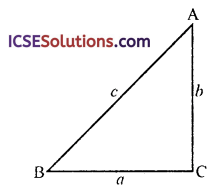Solution:
In right angled ∆ABC(a) a = 6, 6 = 8, then
c² = a² + b² (by pythagoras theorem)
∴ c = $$\sqrt{a^2+b^2}$$
= $$\sqrt{(6)^2+(8)^2}=\sqrt{36+64}$$
= $$\sqrt{100}$$ = 100
∴ c = 10

(b) c = 25, a = 24
∵ c² = a² + b²
⇒ (25)² = (24)² + b²
⇒ 625 = 526 + b²
⇒ b² = 625 – 576 = 49 = (7)²
∴ b = 7

(c) c = 13, b = 5
∵ c² = a² + b²
⇒ (13)² = a² + (5)²
⇒ 169 = a² + 25
⇒ a² = 169 – 25
⇒ a² = 144 = (12)²
∴ a = 12

(d) a = 10, c = 21
∵ c² = a² + b²
∴ (21)² = (10)² + b²
⇒ 441 = 100 + b²
⇒ b² = 441 – 100
⇒ b² = 314 ⇒ b = $$\sqrt{341}$$

(e) a = 9, b = 9
∵ c² = a² + b²
⇒ (9)² + (9)² = 81 + 81 = 162
= 2 x 81 = ($$\sqrt{2}$$ x 9)²
= (9$$\sqrt{2}$$)²
c = 9$$\sqrt{2}$$Question 2.
A rectangular field is 30 m by 40 m. What distance is saved by walking diagonally across the field.
Solution:
In the rectangular field, length (l) = 40 m
BD is its diagonal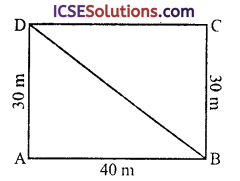∴ In right ∆ABD,
(Pythagoras Theorem)
= (40)² + (30)² = 1600 + 900
= 2500 = (50)²
∴ BD = 50 m
Actual saving = (AB + AD) – BD
= (40 + 30 – 50) = 20 m

Question 3.
A man travels 7 km due north, then goes 3 km due east and then 3 km due south. How far is he from his starting point?
Solution:
A man travels 7 km due north and then 3 km east then 3 km due southOA = 7 km, AB = 3 km, BC = 3 km
Join OC and draw CD || AB
Then OD = AO – AD
= 7 – 3 = 4 km
Now in right ∆OCD,
OC² = OD² + CD² (Pythagoras Theorem)
= (4)² + (3)²
= 16 + 9 = 25 = (5)²
∴ OC = 5
∴ He is 5 km from the starting point.

Question 4.
The diagonals of a rhombus are 12 cm and 9 cm long. Calculate the length of one side of the rhombus.
Solution:
In a rhombus ∆BCD, its diagonals AC and BD bisect each other at right angles at O and AC = 12 cm, BD = 9 cm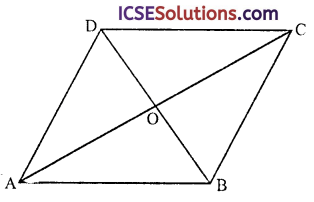∴ AO = OC = $$\frac { 12 }{ 2 }$$ = 6 cm
and BO = OD = $$\frac { 9 }{ 2 }$$ cm = 4.5 cm
Now in right ∆AOB,
AB² = AO² + BO² (Pythagoras Theorem)
= (6)² + (4.5)² = 36 + 20.25
= 56.25 = (7.5)²
∴ AB = 7.5
Hence length of each side = 7.5 cmQuestion 5.
(a) In a right-angled triangle ABC it is given that the hypotenuse, AC = 2.5 cm, and the side AB = 1.5 cm. Calculate the side BC.
(b) AD is drawn perpendicular to BC, base of an equilateral triangle ABC. Given BC = 10 cm, find the length of AD, correct to 1 place of decimal.
Solution:
(a) In right angled ∆ABC, ∠B = 90°
∴ AC² = AB² + BC² (Pythagoras Theorem)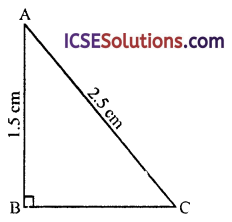⇒ (2.5)² = (1.5)² + BC²
⇒ 6.25 = 2.25 + BC²
⇒ BC² = 6.25 – 2.25 = 4.00 = (2)²
∴ BC = 2 cm

(b) In an equilateral ∆ABC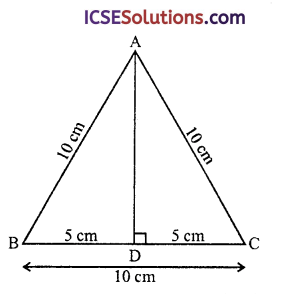∴ D is mid-point of BC
BC = 10 cm
∴ BD = $$\frac { 10 }{ 2 }$$ = 5 cm
and AB = AC = BC = 10 cm
Now in right ∆ABD
AB² = AD² + BD² (Pythagoras Theorem)
⇒ AD² = 10² – 5²
⇒ AD² = 100 – 25 = 75 = 5 x 25
AD = $$\sqrt{5 \times 25}=5 \sqrt{3}$$ cm = 8.7 cm

Question 6.
ABC is right-angled triangle. Angle ABC = 90°, AC = 25 cm and AB = 24 cm. Calculate the area of a ∆ABC.
Solution:
In right ∆ABC, ∠ABC = 90°
AC = 25 cm and AB = 24 cm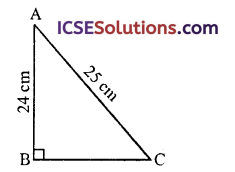∴ AC² = AB² + BC² (Pythagoras Theorem)
⇒ (25)² = (24)² + BC²
⇒ 625 = 576 + BC²
⇒ BC² = 625 – 576 = 49 = (7)²
∴ BC = 7 cm
Now area of ∆ABC = $$\frac { 1 }{ 2 }$$ BC x AB (∆ = $$\frac { 1 }{ 2 }$$ Base x Altitude)
= $$\frac { 1 }{ 2 }$$ x 7 x 24 = 84 cm²

Question 7.
A ladder 13 m long rests against a vertical wall. If the foot of the ladder is 5 m from the foot of the wall, find the distance of the other end of the ladder from ground.
Solution:
Let AC be ladder and its foot C is 5 away from the wall AB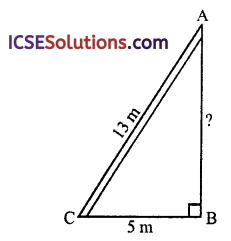AC = 13 m and BC = 5 m
∴ AC² = AB² + BC²
⇒ (13)² = (AB)² + (5)²
⇒ 169 = AB² + 25
⇒ AB² = 169 – 25 = 144
⇒ AB² = (12)² ⇒ AB = 12
∴ Height of the wall = 12 m

Question 8.
Use the given information to make a neat diagram of the figure having given properties and write down the name of the figure.
In triangle ABC, AB² = BC² + Ac² and AC = 2 BC.
Solution:
We are given, in the triangle ABC,
AB² = BC² + AC²
and AC = 2BC
∵ AB² = BC² + AC²
∴ ∆ABC is a right angled at C(i) Draw a line segment BC of some length
(ii) At C, draw a ray CX making 90° at BC and cut off CA = 2BC
Join AB,
Then ∆ABC is the required triangle

Question 9.
In the figure, ABCD represents a quadrilateral in which AD = 13 cm, DC = 12 cm, BC = 3 cm, and ∠ABD = ∠BCD = 90°. Calculate the length of AB.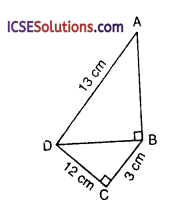Solution:
In the figure ABCD is a quadrilateral in which AD = 13 cm, DC = 12 cm, BC = 3 cm and ∠ABD = ∠BCD = 90°
In right ∆BCD
BD² = CD² + BC² (Pythagoras Theorem)
= (12)² + (3)² = 144 + 9 = 153
Now in right ∆ABD,
(13)² = AB² – 153 ⇒ 169 = AB² + 153
⇒ AB² = 169 – 153 = 16 = (4)²
∴ AB = 4 cm

Question 10.
Which of the triangles whose sides are given below are right angled?
(i) 4 cm, 5 cm, 6 cm
(ii) 1.2 cm, 3.7 cm, 3.5 cm
(iii) 4 cm, 9.6 cm, 10.4 cm
(iv) 2.2 cm, 3.3 cm, 4.4 cm
Solution:
We know that in a right angled triangle,
(Hypotenuse)² = Sunt of squares of other two sides and hypotenuse is the longest side i.e. If square of longest side = Sum of the square of other two sides
Then the triangle is a right angled
(Converse of Pythagoras Theorem)
(a) 4 cm, 5 cm, 6 cm
Here longest side = 6 cm
Now (6)² = 36
and sum of squres of other two sides
= (4)² + (5)² = 16 + 25 = 41
∵ (36) ≠ 41
∴ It is not a right angled triangle

(b) 1.2 cm, 3.7 cm, 3.5 cm Here longest side = 3.7
∴ (3.7)² = 13.69
and (1.2)² + (3.5)² = 1.44 + 12.25 = 13.69
∵ 13.69 = 13.69
∴ It is a right angled triangle(c) 4 cm, 9.6 cm, 10.4 cm
Here longest side = 10.4 cm
∴ (10.4)² = 108.16
and (4)² + (9.6)² = 16.00 + 92.16 = 108.16
∵ 108.16 = 108.16
∴ It is a right angled triangle

(d) 2.2 cm, 3.3 cm, 4.4 cm
Here longest side = 4.4 cm
∴ (4.4)² = 19.36
and (2.2)² + (3.3)² = 4.84 + 10.89 = 15.73
∵ 19.36 ≠ 15.73
∴ It is not a right angled tirangle

Question 11.
In the figure, find the distance of D from A, unit of length is 1 cm, (AB = 2. BC = 4, CD = 2).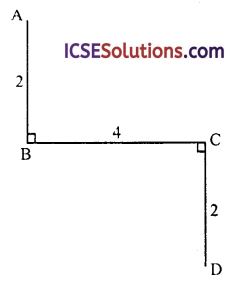Solution:
In the figure, AB = 2 units, BC = 4 units, CD = 2 units
and ∠ABC = ∠BCD = 90°
Join AD which intersects BC at EIn ∆ABE and ∆CDE
AB = CD (each equal to 2 units)
∠AEB = ∠DEC (vertically opposite angle)
and ∠ABE = ∠DCE (each = 90°)
∴ ∆ABE ≅ ∆CDE (ASA axiom)
∴ AE = ED (c.p.c.t.)
and BE = EC = $$\frac { 4 }{ 2 }$$ = 2 units
Now in right ∆ABE
AE² = AB² + BE²
= (2)² + (2)² (Pythagoras Theorem)
= 4 + 4 = 8 = (2$$\sqrt{2}$$)²
∴ AE = 2$$\sqrt{2}$$ units
AD = 2AE = 2 x 2$$\sqrt{2}$$ = 4$$\sqrt{2}$$ units
= 4 (1.414) = 5.656 = 5.66 cm

Question 12.
The sides of a right-angled triangle containing the right angle are 5x and (3x – 1) cm. If the area of the triangle be 60 cm², calculate the lengths of the sides of the triangle.
Solution:
In right angled ∆ABC,
AB = 5x cm and BC = (3x – 1) cm
Area of ∆ABC = 60 cm²Either x – 3 = 0, then x = 3
or 3x + 8 = 0 then 3x = – 8 ⇒ x = $$\frac { -8 }{ 3 }$$
Which is not possible being negative
∴ x = 3
∴ AB = 5x = 5 x 3 = 15
BC = 3x – 1 = 3 x 3 – 1 = 9 – 1 = 8
Now in right ∆ABC
AC² = AB² + BC² (Pythagoras Theorem)
= (15)² + (8)² = 225 + 64
= 289 = (17)²
∴ AC= 17
Hence sides are 15 cm, 8 cm and 17 cm

Question 13.
If the figure, it is given that AB = BC = 25 m. If AE = 7 m, and CD = 24 m, find the length of DE and also show that triangle ABE and triangle BDC are congruent.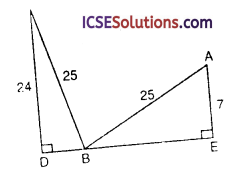Solution:
In the given figure,
AB = BC = 25 m.
If AE = 7 m and CD = 24 m
In right ∆BCD
BC² = CD² + BD² (Pythagoras Theorem)
⇒ (25)² = (24)² + DB² ⇒ DB² = 576 + DB²
⇒ DB² = 625 – 576 = 49 = (7)²
∴ DB = 7m
Similarly in right ∆ABE
AB² = AE² – BE²
⇒ (25)² = (7)² + BE² ⇒ 625 = 49 + BE²
⇒ BE² = 625 – 49 = 576 = (24)²
∴ BE = 24 m
∴ DE = DB + BE = 7 + 24 = 31 m
Now in ∆ABE and ∆ABCD
AB = BC (each = 25 m)
AE = DB (each = 7 m)
BE = CD (each = 24 m)
∆ABE ≅ ∆BCD (SSS axiom)
Hence proved.

Question 14.
A ladder rests against a vertical wall at a height of 12 m from the ground with its foot at a distance of 9 m from the wall on the ground. If the foot of the ladder is shifted 3 m away from the wall, how much lower will the ladder slide down?
Solution:
Let AB be the ladder and AC be the wall, then
AC = 12 m and BC = 9 m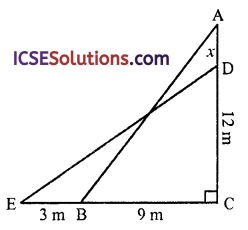∴ AB² = Ac² + BC² (Pythagoras Theorem)
= (12)² + (9)²
= 144 + 81 = 225 = (15)² = 15 m
∴ Length of ladder = 15 m
Again DE is the ladder = AB = 15 m
and on shifting its foot 3 m away from the foot of the wall,
The distance EC = 9 + 3 = 12 m
Now in right ∆DEC
DE² = EC² + DC²
(15)² = (12)² + DC²
⇒ 225 = 144 + DC²
⇒ DC² = 225 – 144
⇒ DC² = 81 = (9)²
∴ DC = 9 m
∴ AD = AC – DC = 12 – 9 = 3 m 3 m
∴ 3 m is lower will be ladderQuestion 15.
AD is an altitude of a AABC and AD is 12 cm, BD = 9 cm and DC = 16 cm long respectively. Prove that the angle BAC is a right angle.
Solution:
Given : In ∆ABC, AD ⊥ BC
AD = 12 cm, BD = 9 cm and DC = 16 cmTo prove : ∠BAC = 1 rt angle
Proof: In right ∆ABD,
AB² = AD² + BD² (Pythagoras Theorem)
= (12)² + (9)² = 144 + 81 = 225 = (15)²
∴ AB = 15 cm
Similarly in right ∆ACD,
= (12)² + (16)² = 144 + 256 = 400 = (20)²
∴ AC = 20 cm
and BC = BD + DC = 9 + 16 = 25 cm
Now (BC)² = (25)² = 625
and AB² + Ac² = (15)² + (20)²
= 225 + 400 = 625
∴ AB² + AC² = BC²
∴ ∆ABC is a right angle, right angled at
∴ ∠BAC = 90°
Hence proved.

Question 16.
The shortest distance AP from a point A to a straight line QR is 12 cm, and Q, R are 15 cm and 20 cm distance from A on opposite sides of AP. Prove that QAR is a right angle.
Solution:
Given : In ∆AQR, AP ⊥ QR
AP = 12 cm, AQ = 15 cm and AR = 20 cmTo prove : ∠QAR is a right angle
Proof: ∵ AP ⊥ QR
∴ In right ∆APQ
AQ² = AP² + QP²
(15)² = (12)² + QP² (Pythagoras Theorem)
∴ 225 = 144 + QP² ⇒ QP² = 225 – 144 = 81
⇒ QP² = (9)² ⇒ QP = 9 cm
Similarly in right ∆APR
AR² = AP² + PR²
⇒ (20)² = (12)² + PR² ⇒ 400 = 144 + PR²
⇒ PR² = 400 – 144 = 256 = (16)²
∴ PR = 16 cm
Now QR = QP + PR = 9 + 16 = 25 cm
In ∆QAR,
Now (QR)² = (25)² = 625
and AQ² + AR² = (15)² + (20)² = 225 + 400 = 625
∵ QR² = AQ² + AR²
∴ ∆AQR is a right angled triangle
and ∠QAR = 1 right angle
Hence proved.

Question 17.
In the figure, PT is an altitude of the triangle PQR in which PQ = 25 cm, PR = 17 cm, PT = 15 cm, and QR = x cm. Calculate x.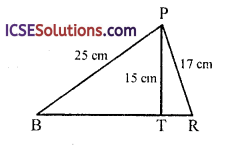Solution:
In the figure, PQR is a triangle in which PT ⊥ BR
PQ = 25 cm, PR = 17 cm, PR = 15 cm, QR = x cm
In right ∆PQR
PQ² = PT² + QT² (Pythagoras Theorem)
⇒ (25)² = (15)² + QT²
⇒ 625 = 225 + QT²
⇒ QT² = 625 – 225 = 400 = (20)²
∴ QT = 20 cm
Similarly in right ∆PTR,
PR² = PT² + TR²
(17)² = (15)² + (TR)²
⇒ TR² = 289 – 225 + TR²
⇒ TR² = 289 – 225 = 64 = (8)²
∴ TR = 8 cm
Now QR = x = QT + TR
= 20 + 8 = 28 cm

Question 18.
In the figure, AB = 8 cm, BC = 6 cm, AC = 3 cm, and the angle ADC = 90°. Calculate CD.Solution:
In the figure, AB = 8 cm, BC = 6 cm, AC = 3 cm and ∠ABC = 90°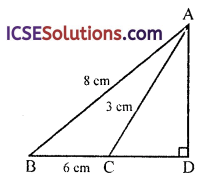Let CD = x
∴ BD = (6 + x)
Now in right ∆ABD
AB² = BD² + AD² (Pythagoras Theorem)
⇒ (8)² = (6 + x)² + AD² ⇒ AD² = (8)² – (6 + x)²
AD² = 64 – (6 + x)² … (i)
Similarly in right ∆ACD,
⇒ (3)² = x² + AD² ⇒ 9 = x² + AD²
⇒ AD² = 9 – x² … (ii)
From (i) and (ii)
64 – (6 + x)² = 9 – x²
64 – (36 + 12x + x²) = 9 – x²
⇒ 64 – 36 – 12x – x² = 9 – x²
28 – 12x – x² + x² = 9
– 12x = 9 – 28 = – 19
⇒ x = $$\frac { -19 }{ -12 }$$ = $$\frac { 19 }{ 12 }$$
∴ CD = $$\frac { 19 }{ 12 }$$ = 1$$\frac { 7 }{ 12 }$$

Question 19.
In the figure, the angle BAC is a right angle and AD is perpendicular to BC; AB = 4 cm, AC = 3 cm and BD = x. Calculate x.Solution:
In right ∆ABC, ∠A = 90°
AB = 4, AC = 3, BD = x
In right ∆ABC
BC² = AB² + AC² (Pythagoras Theorem)
= (4)²+ (3)²= 16 + 9 = 25
= (5)²
∴ BC = 5
BD = x
∴ DC = (5 – x)
Now in right ∆ABD,
= (4)² – x² = 16 – x² … (i)
and in right ∆ACD
= (3)² – (5 – x)²
= 9 – (5 – x)² … (ii)
From (i) and (ii),
16 – x² = 9 – (5 – x)²
⇒ 16 – x² = 9 – (25 – 10x + x²)
⇒ 16 – x² = 9 – 25 + 10x – x²
⇒ 16 – x² – 9 + 25 – 1
⇒ – 10x + x² = 0 ⇒ 10x + 32 = 0
⇒ x = $$\frac { 32 }{ 10 }$$ = 32

Question 20.
In the figure, the angle BAD and ADC are right angles and AX is parallel to BC. If AB = BC = 5 cm, and DC = 8 cm. Calculate the area of ABCX.Solution:
In the figure, AB = BC = 5 cm
∴ AX = BC = 5 cm
CD = 8 cmAX || BC and ∠ADC = ∠DAB = 90°
∵ AX || BC
∴ AB = XC = 5 cm
∴ DX = 8 – 5 = 3 cm